How Cheenta works to ensure student success?
Explore the Back-Story

# Test of Mathematics Solution Subjective 46 - Number of Onto FunctionsThis is a Test of Mathematics Solution Subjective 46 (from ISI Entrance). The book, Test of Mathematics at 10+2 Level is Published by East West Press. This problem book is indispensable for the preparation of I.S.I. B.Stat and B.Math Entrance.

Also visit: I.S.I. & C.M.I. Entrance Course of Cheenta

## Problem

A function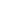from set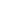into set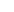is a rule which assigns each element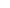in, a unique (one and only one) element (denoted by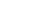in. A function of set fromintois called an onto function, if for each element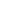inthere is some elementin, such that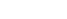. Now suppose that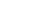{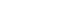} and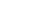{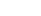}. Determine the total number of onto functions ofinto.

## Sequential Hints

(How to use this discussion: Do not read the entire solution at one go. First, read more on the Key Idea, then give the problem a try. Next, look into Step 1 and give it another try and so on.)

### Key Idea

This is the generic use case of Inclusion-Exclusion Principle. Read on this from Wikipedia

### Step 1

1 may map to 1, 2, or 3 (3 choices). For each of these three choices, may map to 1, 2, or 3 (again three choices. Hence 3 choices again. How many functions are possible in total? Can you delete the functions which are not onto from the total number of functions?

Try the problem with this hint before looking into step 2. Remember, no one learnt mathematics by looking at solutions.

The total number of functions is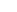since there are 3 output choices for each of the n input choices. Now let us count the functions which are not onto.

How many functions are there such that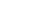has no preimage? (that is nothing is going to 1). There aresuch functions as each of the n elements of A has 2 choices. Similarly, count the cases where nothing goes to 2 (more cases) and nothing goes to 3 (more cases).

Delete these 'not onto' cases (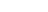) from total number of cases ().

But we have double deleted the case where nothing goes to 1 'and' nothing goes to 2. This is the case where everything goes to 3. Only one such function is there: f(x) = 3.

Similarly, we have double deleted the case where nothing goes to 2 'and' nothing goes to 3. This is the case where everything goes to 1. Only one such function is there: f(x) = 1.

Similarly, we have double deleted the case where nothing goes to 1 'and' nothing goes to 3.

This is the case where everything goes to 2. Only one such function is there: f(x) = 2. Since we do not want to double delete something, we add back these three cases.

Thus the final answer is: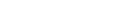This is a Test of Mathematics Solution Subjective 46 (from ISI Entrance). The book, Test of Mathematics at 10+2 Level is Published by East West Press. This problem book is indispensable for the preparation of I.S.I. B.Stat and B.Math Entrance.

Also visit: I.S.I. & C.M.I. Entrance Course of Cheenta

## Problem

A functionfrom setinto setis a rule which assigns each elementin, a unique (one and only one) element (denoted byin. A function of set fromintois called an onto function, if for each elementinthere is some elementin, such that. Now suppose that{} and{}. Determine the total number of onto functions ofinto.

## Sequential Hints

(How to use this discussion: Do not read the entire solution at one go. First, read more on the Key Idea, then give the problem a try. Next, look into Step 1 and give it another try and so on.)

### Key Idea

This is the generic use case of Inclusion-Exclusion Principle. Read on this from Wikipedia

### Step 1

1 may map to 1, 2, or 3 (3 choices). For each of these three choices, may map to 1, 2, or 3 (again three choices. Hence 3 choices again. How many functions are possible in total? Can you delete the functions which are not onto from the total number of functions?

Try the problem with this hint before looking into step 2. Remember, no one learnt mathematics by looking at solutions.

The total number of functions issince there are 3 output choices for each of the n input choices. Now let us count the functions which are not onto.

How many functions are there such thathas no preimage? (that is nothing is going to 1). There aresuch functions as each of the n elements of A has 2 choices. Similarly, count the cases where nothing goes to 2 (more cases) and nothing goes to 3 (more cases).

Delete these 'not onto' cases () from total number of cases ().

But we have double deleted the case where nothing goes to 1 'and' nothing goes to 2. This is the case where everything goes to 3. Only one such function is there: f(x) = 3.

Similarly, we have double deleted the case where nothing goes to 2 'and' nothing goes to 3. This is the case where everything goes to 1. Only one such function is there: f(x) = 1.

Similarly, we have double deleted the case where nothing goes to 1 'and' nothing goes to 3.

This is the case where everything goes to 2. Only one such function is there: f(x) = 2. Since we do not want to double delete something, we add back these three cases.

Thus the final answer is:### Knowledge Partner